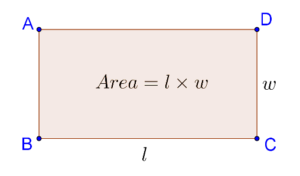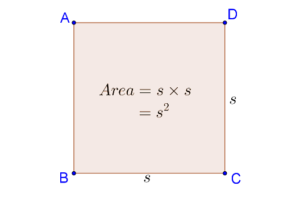# Basic Area Concepts

Go back to  'Triangles-Quadrilaterals'

You know that the area of a geometrical figure represents its planar extent. Calculating the areas of planar geometrical figures is an important practical problem. For example, in the construction of a house, the floor area has a crucial role. Farmers routinely have to deal with issues related to the areas of their lands. In building ultra-fast computers, designers have to fit a very high number of electronic components in extremely small chip areas. Clearly, area problems occur in a variety of situations.

We note the following important facts about areas:

1. Two congruent figures will have the same area. This should be obvious, since two congruent figures can be exactly superimposed over each other.
2. Two non-congruent figures can have the same area. In other words, if two figures have the same area, you cannot say that they are congruent. As a simple example, consider a rectangle with sides 8 cm and 2 cm, and a square with each side equal to 4 cm. Both of these non-congruent figures will have the same area.
3. The area of a rectangle with length l and width w is A = l ×w:i

4. In the case of a square with side s, the area will be A = s × s = s2: